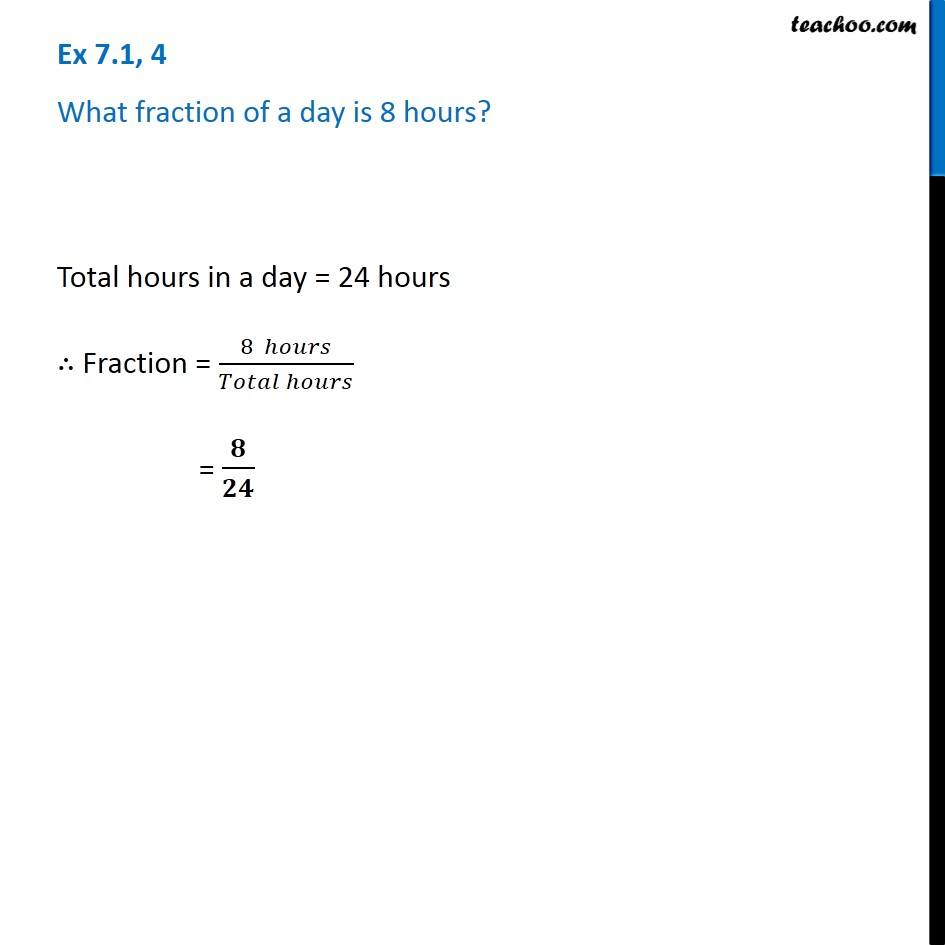1. Chapter 7 Class 6 Fractions
2. Concept wise
3. Finding fraction

Transcript

Ex 7.1, 4 - Chapter 7 Class 6 Fractions - NCERT Book What fraction of a day is 8 hours? Total hours in a day = 24 hours ∴ Fraction = (8 hours) / (Total hours) = 8/24